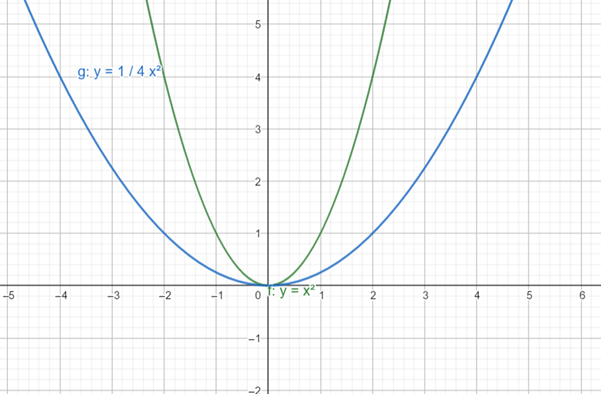# To graph: the function f ( x ) = 1 4 x 2 using the graph of a standard function and then applying transformations.### Precalculus: Mathematics for Calcu...

6th Edition
Stewart + 5 others
Publisher: Cengage Learning
ISBN: 9780840068071### Precalculus: Mathematics for Calcu...

6th Edition
Stewart + 5 others
Publisher: Cengage Learning
ISBN: 9780840068071

#### Solutions

Chapter 2.5, Problem 33E
To determine

## To graph: the function f(x)=14x2 using the graph of a standard function and then applying transformations.

Expert Solution

### Explanation of Solution

Given information:

the function is f(x)=14x2

Graph:

To plot the graph of f(x)=x2 ,use the plotting points method, substitute different value of x on f(x)=x2 to determine corresponding value of f(x) .

The table is thus obtained as:

 x -3 -2 -1 1 2 3 y 9 4 1 1 4 9

now plot each point of x along the horizontal axis and the corresponding value of f(x)=y along vertical axis and connect each point to form a smooth curve.

The graph of f(x)=x2 is obtained as:Now, the graph of f(x)=14x2 is the graph of f(x)=x2 shrinking vertically by a factor of 14 .

The graphof f(x)=14x2 is obtained as:Interpretation:

From the above graph it can be obtained that the graph of f(x)=14x2 is obtained by shrinkingthe graph of f(x)=x2 vertically by a factor of 14 .

### Have a homework question?

Subscribe to bartleby learn! Ask subject matter experts 30 homework questions each month. Plus, you’ll have access to millions of step-by-step textbook answers!